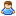1.This forum is obsolete and read-only. Feel free to contact us at support.keenswh.com

# Inaccurate Gravity Vector

Discussion in 'Programming Questions and Suggestions' started by Flaschenhaxel, Mar 19, 2019.

This last post in this thread was made more than 31 days old.
1. ### FlaschenhaxelTrainee Engineer

Messages:
2
I am currently working on a script that searches for the nearest point directly over a given point(T) over the surface of the Planet, so I can drop a Crate with parachutes on that given point.
The Problem is that i do not understand the new IntergridCommunicationSystem and I need T on the Surface as well as the Gravity Vector at T on a ship not connected to the grid at T. I tried to get both via .GetPosition() and .GetNaturalGravity from a RemoteControl and display the X,Y,Z Components on a LCD screen, Copy them and paste Them in the Programming Block on the ship. After tests it shows that on short ranges it is accurate, the higher up i go the further i move from the base, seen on the X,Y plane. thats a problem as i plan to position a ship on the ege of the planets gravitywell.
I think the reason why is that the LCD display just shows whole Numbers no decimal places and so the Gravity Vector i get might be inaccurate.
Is there a way to show a more accurate representation of the Gravity Vector on the LCD panel, or does someone know a better way to transmit the Informations needed?

Code:
```public void Main()

{

//Setting up Variables
IMyRemoteControl RC = GridTerminalSystem.GetBlockWithName("Remote Control") as IMyRemoteControl;
var lcd = GridTerminalSystem.GetBlockWithName("LCD Panel") as IMyTextPanel;
var lcd2 = GridTerminalSystem.GetBlockWithName("LCD Panel 2") as IMyTextPanel;
//Getting Target Coordiantes and putting them on the LCD
double x = Math.Floor(RC.GetPosition().GetDim(0));
double y = Math.Floor(RC.GetPosition().GetDim(1));
double z = Math.Floor(RC.GetPosition().GetDim(2));
string str = x.ToString() + ", " + y.ToString() + ", " + z.ToString();
lcd.WritePublicText(str);
lcd.ShowTextureOnScreen();
lcd.ShowPublicTextOnScreen();
// and the same with the Gravity Vector
double a = Math.Floor(RC.GetNaturalGravity().GetDim(0));
double b = Math.Floor(RC.GetNaturalGravity().GetDim(1));
double c = Math.Floor(RC.GetNaturalGravity().GetDim(2));
string Grav = a.ToString() + ", " + b.ToString() + ", " + c.ToString();
lcd2.WritePublicText(Grav);
lcd2.ShowTextureOnScreen();
lcd2.ShowPublicTextOnScreen();
}```
The code for T and the Gravity Vector
Code:
```Vector3D BaseGPS = new Vector3D(-51326, -28395, 16533); // Coordiantes of T
IMyRemoteControl RemoteControl = GridTerminalSystem.GetBlockWithName("Remote Control") as IMyRemoteControl;
IMyTextPanel LCD = GridTerminalSystem.GetBlockWithName("Text panel") as IMyTextPanel;
Vector3D GV = new Vector3D(8,4,-3); // Gravity Vector

Vector3D CurrentPos = RemoteControl.GetPosition();
Vector3D q = CurrentPos-BaseGPS;
double p = q.X * GV.X + q.Y * GV.Y + q.Z * GV.Z; //finding out Space engineers doesnt understand a normal scalar
double v = GV.Length();										 //Calculation of the nearest point above T step by step to minimize errors
double a = p/(v*v);
Vector3D M;
M.X = a * GV.X;
M.Y = a * GV.Y;
M.Z = a * GV.Z;
Vector3D Destination = BaseGPS + M;

//Displaying Coordinates of the nearest point above T on the LCD to add them manualy to my GPS
double x = Math.Floor(Destination.GetDim(0));
double y = Math.Floor(Destination.GetDim(1));
double z = Math.Floor(Destination.GetDim(2));
string Dest = x.ToString() + ", " + y.ToString() + ", " + z.ToString();

LCD.WritePublicText(Dest);
LCD.ShowTextureOnScreen();
LCD.ShowPublicTextOnScreen();

```
and the code to find the nearest point above T

I know the math i am doing but I don't know how to code exept what i learned in a beginners course and what i thought my self yesterday.

2. ### MalwareMaster Engineer

Messages:
9,867
You say the LCD just shows whole numbers, but you are doing that yourself by flooring the destination.X, Y and Z. By doing that you're just getting the whole numbers.

3. ### FlaschenhaxelTrainee Engineer

Messages:
2
Thank you very much. This fixed it for me.